# Performance improvement of wind turbine with squirrel-cage induction generator by static synchronous compensator and hybrid energy storage system

Performance improvement of wind turbine with squirrel-cage induction generator by static synchronous compensator and hybrid energy storage system

Lei LiuShengtie Wang

College of Energy and Power Engineering, Inner Mongolia University of Technology, Hohhot 010051, China

Corresponding Author Email:
liu_lei1982@sohu.com
Page:
293-312
|
DOI:
https://doi.org/10.3166/EJEE.19.293-312
|
Accepted:
|
Published:
31 December 2017
| Citation

OPEN ACCESS

Abstract:

Wind turbines with squirrel-cage induction generators (SCIGs) face multiple challenges in power quality and fail-safe operation at grid failures, calling for a combination of reactive power compensation device and energy storage system. Therefore, this paper puts forward a novel control plan based on the static synchronous compensator (STATCOM) and hybrid energy storage system (HESS). Specifically, the STATCOM was combined with series dynamic breaking resistor (SDBR) to mitigate the voltage fluctuation and improve low-voltage ride-through (LVRT) performance; the four-level power allocation was adopted for a parallel hybrid energy storage system based on supercapacitor and LiFePO4 lithium battery, with the aim to rationally allocate the compensation power between the two energy storage devices and balance the fluctuating wind power. Matlab simulation results show that the proposed control plan can significantly reduce the voltage fluctuations, enhance the LVRT performance, and smooth the power fed into the grid. The research findings help to promote the application of STATCOM/ hybrid energy storage technology in wind farms.

Keywords:

low-voltage ride-through (LVRT), squirrel-cage induction generator (SCIG), static synchronous compensator (STATCOM), series dynamic breaking resistor (SDBR), hybrid energy storage system (HESS)

1. Introduction

In recent years, China has seen a boom in the electricity generation from wind energy, a critical type of new energy, thanks to the abundance of wind resources and the maturity of wind power generators. However, the fast development of wind power generation is faced with a series of challenges. The intermittence, stochasticity and time variation of natural winds have negative impacts on the power quality. For instance, the voltage at the point of common coupling (PCC) may fluctuate and flicker if the wind power produced squirrel-cage induction generators (SCIGs) is directly accessed to the grid. The ensuing short-circuiting may suppress the voltage at generator terminal and push up the generator speed. To recover to the pre-failure state, a large amount of reactive power needs to be introduced to the wind turbine.

## 1.pngFigure 1. LVRT requirements of wind turbines in China

According to the LVRT standard of State Grid Corporation of China (SGCC) in Figure 1, the wind turbines should remain connected to the grid when the PCC voltage is as low as 20% of the rated value for 0.625s, and when the PCC voltage reaches 90% of the rated value after the failure has been cleared. Nonetheless, some SCIGs do not support the LVRT. The lack of LVRT function, coupled with the poor power quality, has bottlenecked the wind power generation with SCIGs and harmed the stability of the grid.

The LVRT performance and power quality can be improved through proper injection of reactive power into the grid, which can also stabilize the PCC terminal voltage and prevent voltage flicker. One of the most popular reactive power injection techniques is the static synchronous compensator (STATCOM). As the key to the fundamental flexible alternating current transmission system (FACTS), the STATCOM is connected in parallel to the grid and is equivalent to a controllable source of reactive current. The reactive current from the STATCOM can follow the variation in the load reactive current, and dynamically compensates the reactive power of the grid. Most of the existing control strategies of the STATCOM rely on very expensive devices (Tian et al., 2013; Zhang et al., 2014; Wessels et al., 2013; George and Chacko, 2013).

Besides, the STATCOM, the series dynamic breaking resistor (SDBR) also helps to enhance LVRT performance and power quality. With simple control, cheap cost, high reliability and low failure rate, the SDBR can improve the PCC voltage under grid failure when it is placed between the wind turbine and the grid. Recent reports (Hossain and Ali, 2014; Rashid and Ali, 2014; Soliman et al., 2014; Tang et al., 2010) have demonstrated the effect of the SDBR in enhancing the LVRT of the SCIG. However, the enhancement effect will be reduced when the wind power system works at a low power factor (Zhou, 2012).

Some scholars have combined the STATCOM with energy storage system (Xue et al., 2013; Liu et al., 2016; Esmaili and Nasiri, 2011; Zhang et al., 2012; Ding et al., 2014). The combined system provides both reactive and active powers to the grid, thus reducing the fluctuation of wind power. There are several types of energy storage systems, namely, the single-battery energy storage system (SBESS), the supercapacitor energy storage system (SESS) and the hybrid energy storage system (HESS) which integrates the SBESS and the SESS. Among them, only the HESS can provide high power and high energy at the same time, and effectively smooth the power fluctuation of wind farms. The existing studies on the HESS are too shallow, failing to make best use of the complementarity between the battery and the supercapacitor.

The supercapacitor is widely adopted in wind power systems because of its high power density, long lifecycle and short charge-discharge time. As shown in Table 1, LiFePO4 lithium battery outperforms the other batteries in energy density, lifecycle, safety and cleanness (Wang, 2013). Thus, this type of battery should be integrated with the supercapacitor to form a robust HESS.

Table 1. Performance comparison of four types of batteries

 Type Energy density (Wh/kg) Power density (W/kg) Cycle life Charge-discharge efficiency Environmental protection Safety Lead-acid 35-50 75-300 500-1500 80% bad good LifeO4 150-200 200-315 >2000 95% good well Na/S 150-300 90-230 >4500 90% good well Vanadium 80-130 50-140 13000 80% good well

In light of the above, this paper puts forward a novel control plan for STATCOM with HESS. Specifically, the STATCOM was combined with the SDBR to improve the LVRT performance, and a parallel HESS containing the supercapacitor and the LiFePO4 lithium battery was connected to the direct current (DC) side of the STATCOM via a bidirectional DC/DC converter. Through four-grade power allocation, the compensation power of the two energy storage devices was allocated rationally. Then, the proposed control plan was verified with a 2MW SCIG model on Matlab. The results show that the proposed control plan achieved good LVRT performance, grid stability and power quality.

The remainder of this paper is organized as follows: Section 2 introduces the topology and control structure of wind power system with SCIG and STATCOM/HESS; Section 3 puts forward the control strategy of STATCOM and SDBR; Section 4 gives the control strategy of HESS with a bidirectional DC/DC converter; Section 5 verifies the proposed control plan through simulation.

2. Topology and control structure of wind power system with SCIG and STATCOM/HESS

2.1. System topology

As shown in Figure 2, our wind power system consists of wind turbine with SCIG, STATCOM, SDBR, HESS and the grid. A 180kilovar capacitor bank is connected to the terminal of the SCIG. Two STATCOMs, based on the principle of voltage-source converter, are shunt connected at the PCC to realize dynamic compensation of reactive power. The HESS is composed of four parts, namely, a supercapacitor, a LiFePO4 lithium battery, two charge-discharge DC/DC controllers (C1; C2). The supercapacitor and the LiFePO4 lithium battery are connected in parallel on the AC side through the respective STATCOM, such that the two devices can be configured separately to fulfill the respective power needs. For accurate control of power charge-discharge, C1 and C2 are used to interface the supercapacitor and the LiFePO4 lithium battery, respectively.

## 2.pngFigure 2. Topology of wind power system

2.2. Control structure

The control structure of our wind power system is illustrated in Figure 3 below. The working state of the system is determined by voltage sag detection and hysteresis control. In normal state, the SDBR is cut off and the HESS while STATCOM works normally to balance the fluctuating wind power. In failure state (e.g. instantaneous short-circuiting), the HESS is cut off while the SDBR starts to work. The SDBR can lift the PCC voltage and absorb the active power, while the STATCOM provides the reactive power to recover the pre-failure nominal PCC voltage and rotor speed.

## 3.pngFigure 3. Control structure of wind power system

3. Control strategy of the STATCOM and the SDBR

3.1. Control mechanism

The STATCOM and the SDBR differ in the transient stability in and after failure. The parallel STATCOM provides reactive power rapidly to partially improve the terminal voltage during failure, and quickly recover to the pre-failure state after the failure. The SDBR regulates the active power of wind turbine during the failure, consumes the active power that cannot be transmitted to the grid, and enhances the transient stability of wind turbine by slowly increasing the generator speed and greatly boosting the terminal voltage. Considering the limitations of each control method, the STATCOM and the SDBR are integrated here to regulate the active and reactive powers of wind turbine in and after failure, improve failure ride-through capability, and reduce the STATCOM rating.

The control effect of the combined strategy on terminal voltage is presented in Figure 4, where $\dot{E}$ is grid voltage, Z is line impedance, $\dot{I}_{S}$  is the terminal current of the generator, $\dot{U}_{S}$  is the terminal voltage of the generator, $\dot{I}_{S C}$  is the compensated terminal current of the generator, $\dot{I}_{C}$  is the compensating current, $\dot{U}_{S C}$  is the compensated terminal voltage of the generator, the solid line is the uncompensated voltage vector-graph and the dashed line is the compensated voltage vector-graph. The constant uncompensated grid voltage $\dot{E}$  can be expressed as:

$\dot{E}=\dot{U}_{S}+\dot{I}_{S} \times Z=\dot{U}_{S C}+\dot{I}_{S C} \times Z$  (1)

where Z=R+jX.

## 4.pngFigure 4. The control effect of the combined strategy on terminal voltage

It can be seen from Figure 4 that, when the STATCOM is used alone, the $\dot{U}_{S C}$  is slightly greater than $\dot{U}_{S}$ , and the improvement effect on terminal voltage is not obvious compared with reactive power compensation. After the introduction of the SDBR, the uncompensated and compensated terminal voltages of the generator, denoted as $\dot{U}$  and $\dot{U}_{1}$ , respectively, can be expressed as:

$\dot{U}=\dot{U}_{S}+\dot{I}_{S} \times R_{S D B R}$  (2)

$\dot{U}_{1}=\dot{U}_{S C}+\dot{I}_{S C} \times R_{S D B R}$   (3)

Thanks to the improved power factor, the $\dot{U}$ is greater than $\dot{U}_{1}$. Therefore, the combined strategy requires lower reactive current and reactive power than the pure STATCOM control and demands lower braking resistor than the pure SDBR control to achieve the same terminal voltage of the generator.

3.2. STATCOM control

As shown in Figure 5, the STATCOM control consists of the independent positive/negative sequence control, the dual second-order generalized integrator (DSOGI), and the decoupled current control.

The outer control loops were designed to control the DC voltage and the positive/negative sequences of the voltage at the connection point of the STATCOM. This calls for the DSOGI-based precise sequence separation of the measured voltage into positive- and negative-sequence components (Kong et al., 2012). After the sequence separation, the positive- and the negative-sequences of the voltage were presented as DC values that can be controlled by proportional-integral (PI) controllers. To ensure the safe operation of the STATCOM in its current capability, the reference currents given by the four outer controllers must fall below the maximum STATCOM current, and the negative-sequence reference currents must be transformed into the positive rotating reference frame by a coordinate transformation with twice the grid voltage angle.

## 5.pngFigure 5. Block diagram of the STATCOM control

The current inner loop control was realized by the PI + resonant (PI-R) control. Compared with the traditional PI regulator, the PI-R control can achieve zero steady-state error to both the fundamental DC reference and the resonant AC reference. In this way, the system interference rejection to grid voltage dip was improved, which ensures the accuracy of the integrated strategy (Liu et al., 2016).

The STATCOM control is a typical coupling system. The change of the reactive current iq will cause the change of the active current id and vice versa. The decoupled controller can be expressed as follows:

$\left\{\begin{array}{l}{X_{1}(s)=\left(K_{p}+\frac{K_{i}}{s}+\frac{2 \omega_{c} K_{R} s}{s^{2}+2 \omega_{c} s+\omega_{0}^{2}}\right)\left(i_{d}^{*}-i_{d}\right)} \\ {X_{2}(s)=\left(K_{p}+\frac{K_{i}}{s}+\frac{2 \omega_{c} K_{R} s}{s^{2}+2 \omega_{c} s+\omega_{0}^{2}}\right)\left(i_{q}^{*}-i_{q}\right)}\end{array}\right.$   (5)

The above formulas show the complete decoupling between the active and reactive currents.

The DSOGI was put forward to quickly and accurately acquire the phase and frequency of the positive-sequence fundamental component of grid voltage, thereby ensuring the safe and reliable operation of the grid-side converter and static reactive compensator under unbalanced grid voltage. The DSOGI is capable of locking the phase of the positive fundamental component and detecting the positive and negative fundamental components of the PCC voltage.

The DSOGI algorithm was verified through two simulation tests. In the simulation under single-phase short-circuiting, the short-circuiting occurred at 0.02s and was cleared after 1.025s. The responses of the PCC voltage, phase, error, frequency, positive-sequence voltage and negative-sequence voltage are recorded in Figure 6. In the simulation under unbalanced voltage, the amplitude of single-phase voltage drops to 50% at 0.1s. The responses of the PCC voltage, phase, error, frequency, positive-sequence voltage and negative-sequence voltage are displayed in Figure 7.

## 6.pngFigure 6. Simulated waveforms under single-phase short-circuiting

## 7.pngFigure 7. Simulated waveforms under unbalanced voltage

As shown in the two figures above, the DSOGI phase locked loop (PLL) quickly locked the phase of the positive fundamental component of the PCC voltage, accurately detected the positive and negative fundamental components of the PCC voltage, and achieved zero steady-state error under the single-phase short circuiting and unbalanced voltage.

3.3. SDBR control

Based on hysteretic control, the SDBR control is illustrated in Figure 8. The upper and lower limits of the hysteresis comparator are denoted as VrefH and VrefL, respectively.

Under normal conditions, the bypass switch of the SDBR is closed, and the SDBR is not connected to the grid. When the PCC voltage is below the lower limit VrefL, the hysteresis controller sends out the control signal to open the bypass switch. Then, the current will flow through the inserted breaking resistor in the failure period and the initial phase of post-failure recovery. When the PCC voltage is above the upper limit VrefH, the bypass switch is closed and the system restores to its pre-failure state.

## 8.png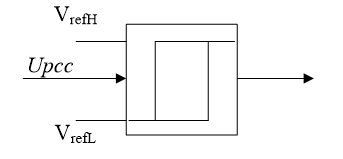Figure 8. Block diagram of the SDBR control

4. Control strategy for HESS with dual DC/DC converters

The HESS control relies on the LiFePO4 lithium battery to compensate low-frequency fluctuation and the supercapacitor to compensate high-frequency fluctuation. Considering the operating frequency range of wind power, 0.5Hz was chosen as the dividing point between high-frequency fluctuation and low-frequency fluctuation.

According to the block diagram in Figure 9, the output active power Pwind of wind turbine is decomposed by a low-pass filter in power smoothing link into smoothing power reference value and hybrid energy storage power reference value.

The hybrid energy storage system allocates power by four devices, namely, a low-pass filter, a fuzzy controller, a power regulation module and a limitation management controller. The low-pass filter splits the demand-generation mismatch into two frequency components; the fuzzy controller regulates the power of power smoothing link, and thus the state of charge (SOC) of energy storage system. According to the SOC of the lithium battery and the supercapacitor, the power allocation between the two energy storage devices can be regulated properly, such as to optimize the SOCs of the lithium battery and the supercapacitor.

## 9.pngFigure 9. Block diagram of the control strategy for HESS

In Figure 9, Kp is the output regulation coefficient of the fuzzy controller, Pnom is the rated power of the hybrid energy storage system, SBat and SSC are the SOCs of the lithium battery and the supercapacitor, respectively, SBat-exp and SSC-exp are the optimal SOCs of the lithium battery and the supercapacitor, respectively, both of which are 0.5. Then, the regulated power Padjust can be expressed as:

$P_{\text {adjust}}=K_{P} \times P_{\text {nom}}$   (6)

For the lithium battery and the supercapacitor, the SOC ranges are 0.2~0.8 and 0.1~0.9, respectively, and the discourse domain ranges are [-0.3, 0.3] and [-0.4, 0.4], respectively. The discourse domain of each input/output variable can be divided into five membership functions: negative big (NB), negative small (NS), zero (ZO), positive small (PS) and positive big (PB). The 25 control rules of the fuzzy controller are listed in Table 2 below.

Table 2. Fuzzy control rules

 U(KP) E1 (ΔSBat) NB NS ZO PS PB E2 (ΔSSC) NB PS PB PB PB PB NS NS NS PS PS PB ZO NS NS ZO PS PS PS NB NS ZO NS PS PB NB NB NB NS NS

Under the charging-discharging capacity of the supercapacitor, the power regulation module regulates the set value of the lithium battery power, and balances the residual capacities of the two energy storage devices, aiming to prevent the lithium battery from receiving the excess charging-discharging power of the supercapacitor and to extend the service life of the hybrid energy storage system. The power regulation value Padjust-sc depends on the SOC of the hybrid energy storage system. Then, the regulated power Padjust' can be expressed as:

$P_{a d j u s t}’=P_{a d j i s t} \times \frac{1}{T s+1}$   (7)

where T is the time constant of the hybrid energy storage system.

When Padjust' is positive and the supercapacitor SOC is greater than 0.5, Padjust-sc is equal to Padjust' if the lithium battery can absorb additional charge; when Padjust' is negative and the supercapacitor SOC is smaller than 0.5, Padjust-sc is equal to Padjust' if the lithium battery can release the additional discharge. The Padjust-sc value is displayed in Table 3.

Table 3. Value analysis of Padjust-sc

The limitation management controller limits the charge-discharge capacity of energy storage device, thereby preventing high-current charge-discharge and extends the service life. As shown in Figure 10, the regulated power of
lithium battery Pbref' is limited by lithium battery limitation. The supercapacitor power PSC can be calculated according to equation (8) by the power of hybrid energy storage Pess and power of lithium battery Pbref'.

Then, the reference power of supercapacitor Pcref is obtained by supercapacitor limitation. Under the difference between PSC and Pcref, the charge-discharge power of hybrid energy storage may not be able to track the set value. Thus, the power should be allocated again. The reference power of lithium battery Pbref is derived from Pess and Pcref, which is limited by lithium battery limitation.

$P_{S C}=P_{\mathrm{ess}}-P_{\text {bref}}’$  (8)

## 10.png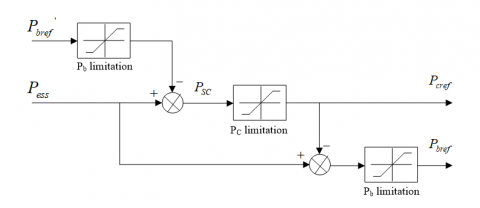Figure 10. Structure of limitation management control

The bidirectional DC/DC converter adopts the double-loop control, consisting of the current inner loop and power outer loop. The high-frequency power component is used to estimate the reference power Pcref of the supercapacitor, which is compared with the actual power of the supercapacitor. The reference current of supercapacitor is given by the outer loop controller and compared with the actual current, thus generating the switching signal for the converter C1. By contrast, the low frequency power component is used to generate the reference power Pbref of the lithium battery, which is compared with the actual power of the lithium battery. The reference current of lithium battery is given by the outer loop controller and compared with the actual current, thus generating the switching signal for the converter C2.

5. Simulation results

5.1 System configuration and parameters

As shown in Figure 11, the proposed system is composed of a wind turbine with SCIG, an STATCOM/HESS, an SDBR and a grid. The parameters of the wind turbine and the STATCOM/HESS are listed in Table 4.

## 11.pngFigure 11. System configuration

Table 4. Parameters of the wind turbine and the STATCOM/HESS

 Wind turbine PN=2MW, RS=0.006pu, XS=0.1413pu, Rr=0.0066pu, Xr=0.0463pu, Xm=4.1338pu, UN=690V, f=50/60Hz,  Jg=5.8078, fc=0.01, p=2 STATCOM/HESS QSTATCOM1=QSTATCOM2=300kvar, Lf=0.99mH, Cdc=0.01016F, Cf=0.06mF, PC=500kW, Pb=390kW, WC=300kWh, Wb=210kWh

5.2. HESS mitigation of active power fluctuation

A noisy wind of different since wave frequencies with a mean speed of 12m/s was applied to the system, in which the initial SOCs of the supercapacitor and the lithium battery are to 0.6 and 0.7, respectively. The power simulation curves on wind speed, output power of wind turbine (Pwind), smoothing power (Pgrid), reference power of LiFePO4 lithium battery (Pb), reference power of supercapacitor (Pc) and regulated power of the fuzzy controller (P’) are shown in Figure 12 below.

## 12.pngFigure 12. Power simulation curves

It can be seen from Figure 12 that the fluctuation of output power was obviously reduced by the low-pass filter. Firstly, the low-pass filter decomposed the reference power of hybrid energy storage into the supercapacitor power and the LiFePO4 lithium battery power. Then, the LiFePO4 lithium battery mitigated low-frequency fluctuation and the supercapacitor mitigated the high-frequency fluctuation. The fuzzy controller generated a certain positive regulation power, as the SOC of hybrid energy storage device was greater than 0.5.

The simulation curves on power fluctuation and the SOC of the lithium battery are shown in Figure 13 and those on the supercapacitor of the supercapacitor are displayed in Figure 14, with the aim to compare the control under power regulation and that without power regulation. It can be seen from the figures that the power of each energy storage device changed constantly to compensate for the wind power fluctuations, and the SOC of each device varied with the charge-discharge. To optimize the SOC of each device with power regualtion, the fuzzy controller generated positive regulation power to change the set value of hybrid energy storage device. Because the SOC of each device was between 0.5 and 0.7, all power regulation of the fuzzy controller was compensated by the supercapacitor. Therefore, the power compensation value and the SOC of the lithium battery was basically unchanged, while the power compensation value of the supercapacitor increased and the SOC of the supercapacitor was close to the optimal value with power regulation.

## 13.pngFigure 13. Simulation curves of LiFePO4 Lithium battery

## 14.pngFigure 14. Simulation curves of supercapacitor

The same simulation was carried out at the initial supercapacitor SOC of 0.3 and the initial lithium battery SOC of 0.38. The resulting power simulation curves of the integrated strategy, the LiFePO4 lithium battery and the supercapacitor are presented in Figures 15, 16 and 17, respectively. It can be seen from these figures that, to optimize the SOC of the hybrid energy storage device with power regulation, the fuzzy controller generated a certain negative regulation power to change the set value of the hybrid energy storage device. Since the SOCs of the supercapacitor and the lithium battery were between 0.5 and 0.3, all power regulation of the fuzzy controller was compensated by the supercapacitor. As a result, the power compensation value of the supercapacitor decreased, and the SOC of the supercapacitor approximated the optimal value with power regulation. The power compensation value and the SOC of the lithium battery dropped slightly due to limitation management control.

## 15.pngFigure 15. Power simulation curves of the integrated strategy

## 16.png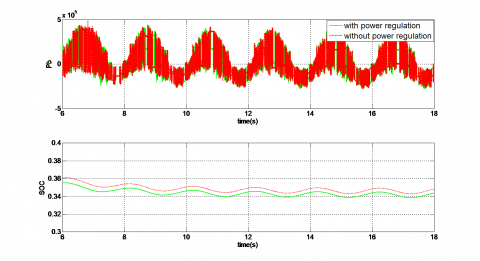Figure 16. Power simulation curves of LiFePO4 Lithium battery

## 17.png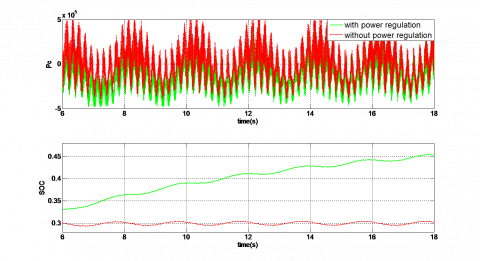Figure 17. Power simulation curves of the supercapacitor

5.3. Voltage stabilization under three-phase short-circuiting

A noisy wind of different since wave frequencies with a mean speed of 12m/s was applied to the system, in which the initial SOCs of the supercapacitor and the lithium battery are to 0.6 and 0.7, respectively. The other simulation parameters are as follows: a three-phase short-circuiting occurred in the 110kV bus at 12s and was cleared after 0.6s, leading to a voltage drop of 20%. The power simulation curves on wind speed, output power of wind turbine (Pwind), smoothing power (Pgrid), reference power of LiFePO4 lithium battery (Pb), reference power of supercapacitor (Pc) and regulated power of the fuzzy controller (P’) are shown in Figure 18 below.

## 18.png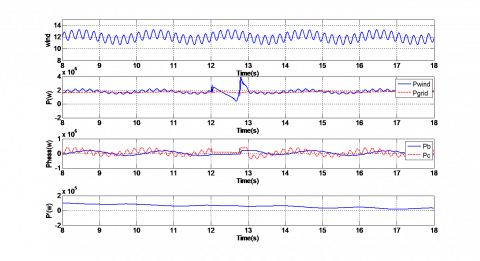Figure 18. Power simulation curves

It can be seen from Figure 18 that the fluctuation of output power was obviously reduced by the low-pass filter. Firstly, the low-pass filter decomposed the reference power of hybrid energy storage into the supercapacitor power and the LiFePO4 lithium battery power. Then, the LiFePO4 lithium battery mitigated low-frequency fluctuation and the supercapacitor mitigated the high-frequency fluctuation. The fuzzy controller generated a certain positive regulation power, as the SOC of hybrid energy storage device was greater than 0.5.

The simulation curves on the PCC voltages with the STATCOM and with STATCOM-SDBR, the rotor speed of the SCIG and the STATCOM-generated reactive powers are presented in Figure 19. Obviously, the reactive power compensation by the STATCOM, coupled with the SDBR, the PCC voltages and the rotor speed recovered to the pre-failure nominal values, and the wind turbine remained connected to the grid. Besides, STATCOM-SDBR had a shorter recovery time and slower SCIG speed increase than the pure STATCOM control. This means the STATCOM-SDBR control can enhance the transient stability, reduce the voltage fluctuation and promote the LVRT capability of wind turbine. The decline in the output reactive power compensation capacity of the STATCOM was also seen from this figure.

## 19.png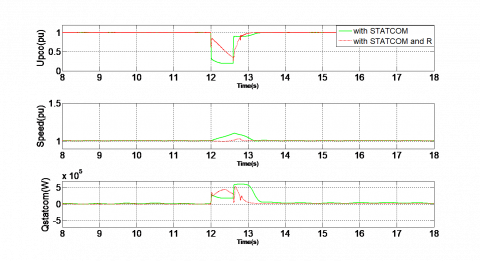Figure 19. Simulation curves under three-phase short-circuiting

The simulation curves on power fluctuation and the SOC of the lithium battery are shown in Figure 20 and those on the supercapacitor of the supercapacitor are displayed in Figure 21, with the aim to compare the control under power regulation and that without power regulation. It can be seen from the figures that the power of each energy storage device changed constantly to compensate for the wind power fluctuations, and the SOC of each device varied with the charge-discharge. To optimize the SOC of each device with power regualtion, the fuzzy controller generated positive regulation power to change the set value of hybrid energy storage device. Because the SOC of each device was between 0.5 and 0.7, all power regulation of the fuzzy controller was compensated by the supercapacitor. Therefore, the power compensation value and the SOC of the lithium battery was basically unchanged, while the power compensation value of the supercapacitor increased and the SOC of the supercapacitor was close to the optimal value with power regulation.

## 20.png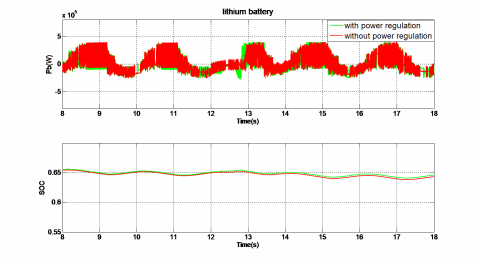Figure 20. Power simulation curves of LiFePO4 Lithium battery

## 21.pngFigure 21. Power simulation curves of the supercapacitor

6. Conclusions

This paper puts forward a novel control plan based on the STATCOM and the SDBR for hybrid energy storage system. Specifically, the STATCOM and the SDBR were adopted to mitigate the voltage fluctuations and improve failure ride-through capability of the wind turbine. The independent controls of positive- and negative- sequences were combined to ensure the safe and reliable STATCOM operation in normal conditions and to enhance the stability of grid voltage. These give birth to a parallel type of hybrid energy storage system based on supercapacitor and LiFePO4 lithium battery, which adopts the four-level power allocation to rationally allocate the compensation power of the two energy storage devices and balance the fluctuating wind power. The proposed control plan was verified through a simulation model for a wind turbine with an SCIG, a STATCOM/HESS and a grid is established in the Matlab/Simulink. The simulation results show that our control plan can boost the power quality and failure ride-through capability of the wind turbine, smooth the power injected grid, and optimize the SOCs of the energy storage devices under power regulation.

Acknowledgement

This work was supported by National Natural Science Foundation of China (51267015).

References

Ding Z., Yang C., Zhang Z., Wang C., Xie S. (2014). A novel soft-switching multiport bidirectional DC-DC converter for hybrid energy storage system. IEEE Trans. Power Electron, Vol. 29, No. 4, pp. 1595-1609. https://doi.org/10.1109/TPEL.2013.2264596

Esmaili A., Nasiri A. (2011). Power smoothing and power ramp control for wind energy using energy storage. 2011 IEEE Energy Conversion Congress and Exposition, pp. 922-927. https://doi.org/10.1109/ECCE.2011.6063870

George S. K., Chacko F. M. (2013). Comparison of different control strategies of STATCOM for power quality improvement of grid connected wind energy system. 2013 International Multi-Conference on Automation, Computing, Communication, Control and Compressed Sensing (iMac4s), pp. 650-655. https://doi.org/10.1109/iMac4s.2013.6526394

Hossain M. K., Ali M. H. (2014). Low voltage ride through capability enhancement of grid connected PV system by SDBR. 2014 IEEE PES T&D Conference and Exposition, pp. 1-5. https://doi.org/10.1109/TDC.2014.6863248

Kong F., Yuan T., Chao Q. (2012). A method of converter power grid synchronization based on second-order generalized integral. Power System Protection and Control, Vol. 40, No. 12, pp. 116-120.

Li F. B., Xie K. G., Zhang X. S. (2013). Control strategy design for hybrid energy storage system based on charge/discharge status of lithium-ion battery. Automation of Electric Power Systems, Vol. 37, No. 1, pp. 70-75.

Liu L., Wang S. T., Tian G. Z. (2016). Grid power quality improvement with STATCOM/HESS for wind turbine with squirrel-cage induction generator. 2016 IEEE 11th Conference on Industrial Electronics and Applications (ICIEA). https://doi.org/10.1109/ICIEA.2016.7604023

Mohamed A., Salehi V., Mohammed O. (2012). Real-time energy management algorithm for mitigation of pulse loads in hybrid microgrids. IEEE Tran. Smart Grid, Vol. 3, No. 4, pp. 1911-1922. https://doi.org/10.1109/TSG.2012.2200702

Rashid G., Ali M. H. (2014). A modified bridge-type fault current limiter for fault ride-through capacity enhancement of fixed speed wind generator. IEEE Transactions on Energy Conversion, Vol. 29, No. 2, pp. 527-534. https://doi.org/10.1109/TEC.2014.2304414

Soliman H., Wang H., Zhou D., Blaabkerg F., Marie M. I. (2014). Sizing of the series dynamic breaking resistor in a doubly fed induction generator wind turbine. 2014 IEEE Energy Conversion Congress and Exposition (ECCE), pp. 1842-1846. https://doi.org/10.1109/ECCE.2014.6953642

Tang F., Liu T., Li X. (2010). Improving transient stability of wind farm consisting of fixed speed induction generator by series connected dynamic braking resistors. Power System Technology, Vol. 34, No. 4, pp. 163-167.

Tian G. Z., Wang S. T., Liu G. C., Wang W. (2013). Mechanism analysis and experimental research on improving performance of wind farm with squirrel-cage induction generators by STATCOM. Power System Technology, Vol. 37, No. 7, pp. 1971-1977.

Wang H. (2013). Study on the lithium battery-supercapacitor hybrid energy storage system and its application in wind power generation. Shang Hai University of Electric Power.

Wessels C., Hoffmann N., Molinas M., Fuchs F. W. (2013). STATCOM control at wind farms with fixed-speed induction generators under asymmetrical grid faults. IEEE Transactions on Industrial Electronics, Vol. 60, No. 7, pp. 2864-2873. https://doi.org/10.1109/TIE.2012.2233694

Xue C., Wang J., Ji Y., Jiang B. (2013). Current decoupling control of STATCOM combined with battery energy storage system. Power System Protection and Control, Vol. 41, No. 6, pp. 43-48.

Zhang K., Li C., Mao C., Lu J., Wang D., Zeng J., Chen X. (2012). Power control of directly-driven wind generation systems with battery/ultra-capacitor hybrid energy storage. Proceedings of the CSEE, Vol. 32, No. 25, pp. 99-108.

Zhang M., Zhang J. Y., Lv M. Y. (2014). Reactive power compensation control of STATCOM based on the improved law of variable structure control. Control Engineering of China, Vol. 21, No. 1, pp. 5-8.

Zhou Z. (2012). Fixed-speed asynchronous wind turbine model and transient stability. Harbin Institute of Technology.